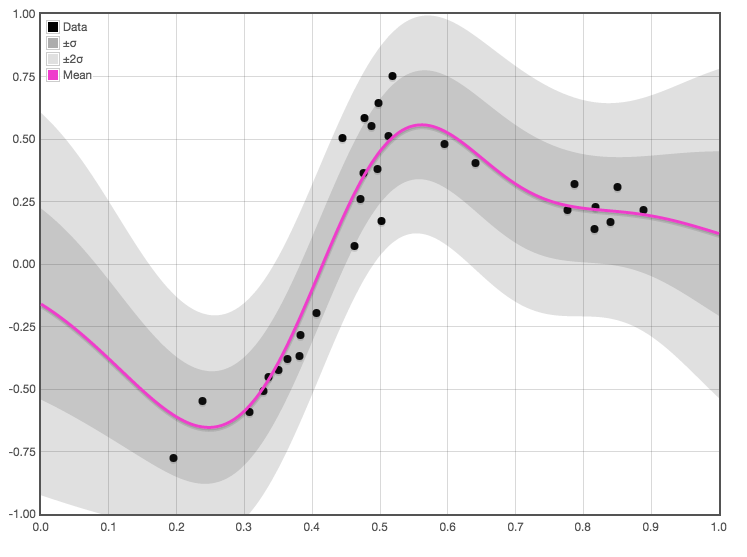# Gaussian process regression

## And classification. And extensions.Gaussian random processes/fields are stochastic processes/fields with jointly Gaussian distributions of observations. While “Gaussian process regression” is not wrong per se, there is a common convention in stochastic process theory (and also in pedagogy) to use process to talk about some notionally time-indexed process and field to talk about ones that have a some space-like index without a special arrow of time. This leads to much confusion, because Gaussian field regression is what we usually want to talk about (although the arrow-of-time can pop up usefully). Hereafter I use “field” and “process” interchangeably, as everyone does in this corner of the discipline.

In machine learning, Gaussian fields are used often as a means of regression or classification, since it is fairly easy to conditionalize a Gaussian field on data and produce a posterior distribution over functions. Because the reuslting regression function can have some very funky and weird posterior distributions, we can think of it as a kind of nonparametric Bayesian inference, although as always with that term we probably want to be careful with it; in fact GP regression typically has parameters.

I would further add that GPs are the crystal meth of machine learning methods, in terms of the addictiveness, and of the passion of the people who use it.

The central trick is using a clever union of Hilbert space tricks and probability to give a probabilistic interpretation of functional regression as a kind of nonparametric Bayesian inference.

Useful side divergence into representer theorems and Karhunen-Loève expansions for give us a helpful interpretation. Regression using Gaussian processes is common e.g. spatial statistics where it arises as kriging. Cressie (1990) traces a history of this idea via Matheron (1963a), to works of Krige (1951).## Lavish intros

I am not the right guy to provide the canonical introduction, because it already exists. Specifically, Rasmussen and Williams (2006). Moreover, because GP regression is so popular and so elegant, there are many excellent interactive introductions online.

This lecture by the late David Mackay is probably good; the man could talk.

There is also a well-illustrated and elementary introduction by Yuge Shi. There are many, many more.

Gaussianprocess.org is a classic.

A Visual Exploration of Gaussian Processes recommends the following:

If you want more of a hands-on experience, there are also many Python notebooks available:

## Brutally quick intro

J. T. Wilson et al. (2021) have a dense and useful perspective. If you are used to this field, they might reboot your perspective. If you are new to the GP area, see the more instructive intros.

A Gaussian process (GP) is a random function $$f: \mathcal{X} \rightarrow \mathbb{R}$$, such that, for any finite collection of points $$\mathbf{X} \subset \mathcal{X}$$, the random vector $$\boldsymbol{f}=f(\mathbf{X})$$ follows a Gaussian distribution. Such a process is uniquely identified by a mean function $$\mu: \mathcal{X} \rightarrow \mathbb{R}$$ and a positive semi-definite kernel $$k: \mathcal{X} \times \mathcal{X} \rightarrow \mathbb{R}$$. Hence, if $$f \sim \mathcal{G} \mathcal{P}(\mu, k)$$, then $$\boldsymbol{f} \sim \mathcal{N}(\boldsymbol{\mu}, \mathbf{K})$$ is multivariate normal with mean $$\boldsymbol{\mu}=\mu(\mathbf{X})$$ and covariance $$\mathbf{K}=k(\mathbf{X}, \mathbf{X})$$.

[…] we investigate different ways of reasoning about the random variable $$\boldsymbol{f}_* \mid \boldsymbol{f}_n=\boldsymbol{y}$$ for some non-trivial partition $$\boldsymbol{f}=\boldsymbol{f}_n \oplus \boldsymbol{f}_*$$. Here, $$\boldsymbol{f}_n=f\left(\mathbf{X}_n\right)$$ are process values at a set of training locations $$\mathbf{X}_n \subset \mathbf{X}$$ where we would like to introduce a condition $$\boldsymbol{f}_n=\boldsymbol{y}$$, while $$\boldsymbol{f}_*=f\left(\mathbf{X}_*\right)$$ are process values at a set of test locations $$\mathbf{X}_* \subset \mathbf{X}$$ where we would like to obtain a random variable $$\boldsymbol{f}_* \mid \boldsymbol{f}_n=\boldsymbol{y}$$.

[…] we may obtain $$\boldsymbol{f}_* \mid \boldsymbol{y}$$ by first finding its conditional distribution. Since process values $$\left(\boldsymbol{f}_n, \boldsymbol{f}_*\right)$$ are defined as jointly Gaussian, this procedure closely resembles that of [the finite-dimensional case]: we factor out the marginal distribution of $$\boldsymbol{f}_n$$ from the joint distribution $$p\left(\boldsymbol{f}_n, \boldsymbol{f}_*\right)$$ and, upon canceling, identify the remaining distribution as $$p\left(\boldsymbol{f}_* \mid \boldsymbol{y}\right)$$. Having done so, we find that the conditional distribution is the Gaussian $$\mathcal{N}\left(\boldsymbol{\mu}_{* \mid y}, \mathbf{K}_{*, * \mid y}\right)$$ with moments \begin{aligned} \boldsymbol{\mu}_{* \mid \boldsymbol{y}}&=\boldsymbol{\mu}_*+\mathbf{K}_{*, n} \mathbf{K}_{n, n}^{-1}\left(\boldsymbol{y}-\boldsymbol{\mu}_n\right) \\ \mathbf{K}_{*, * \mid \boldsymbol{y}}&=\mathbf{K}_{*, *}-\mathbf{K}_{*, n} \mathbf{K}_{n, n}^{-1} \mathbf{K}_{n, *}\end{aligned}

## Kernels

a.k.a. covariance models.

GP regression models are kernel machines. As such covariance kernels are the parameters. More or less. One can also parameterise with a mean function, but (see next) let us ignore that detail for now because usually we do not use them.

## Prior with a mean functions

Almost immediate but not quite trivial .

TODO: discuss identifiability.

## Using state filtering

When one dimension of the input vector can be interpreted as a time dimension we are Kalman filtering Gaussian Processes, which has benefits in terms of speed and hipness.

## On manifolds

I would like to read Terenin on GPs on Manifolds who also makes a suggestive connection to SDEs, which is the filtering GPs trick again.

🏗

## With inducing variables

“Sparse GP”. See Quiñonero-Candela and Rasmussen (2005). 🏗

## By variational inference with inducing variables

See GP factoring.

## Neural processes

See neural processes.

## Observation likelihoods

Gaussian processes need not have a Gaussian likelihood. Classification etc. TBD

## Density estimation

Can I infer a density using GPs? Yes. One popular method is apparently the logistic Gaussian process .

## Approximation with dropout

Unconvincing in practice. See NN ensembles for some vague notes.

## Inhomogeneous with covariates

Integrated nested Laplace approximation connects to GP-as-SDE idea, I think?

e.g. GP-LVM . 🏗